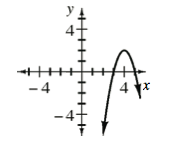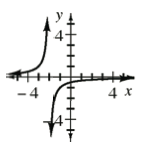### Home > INT3 > Chapter 3 > Lesson 3.1.1 > Problem3-16

3-16.

For each of the functions in problem 3-15, sketch the graph of $y=f(-x)$.

Think about a blank graph. Where are all of the points with a positive $x$-value?
Where are all of the points with a negative $x$-value? How are these points related?